### Write and Solve One-Step Equations

Introduce your students to writing and solving one-step equations with a variety of engaging activities.  When teaching students how to solve equations, you want them to understand that they need to perform the inverse (opposite) operation of the operation that is given.  This will allow them to isolate the variable.

I have created and used all of the resources shown below with my middle school students.  Each of these activities gives my students a variety of opportunities to master writing and solving one-step equations.

This One-Step Equations UNIT RESOURCE BUNDLE includes 40 task cards, 10 error analysis activities and 10 problem solving graphic organizers, 4 homework practice worksheets, 1 maze worksheet, 1 riddle worksheet, 1 coloring page activity (over 125 skills practice and real-world word problems).

Topics included:

💥 Solve & Write Addition Equations

💥 Solve & Write Subtraction Equations

💥 Solve & Write Multiplication Equations

💥 Solve & Write Division Equations

The following math activities are perfect for warm-ups, cooperative learning, spiral review, math centers, assessment prep and homework.  There is a printed and digital version for each activity.

This unit resource bundle includes:

Each page has a real-world word problem that is solved incorrectly. Students have to identify the error, provide the correct solution and share a helpful strategy for solving the problem. Print or use the digital version of this activity.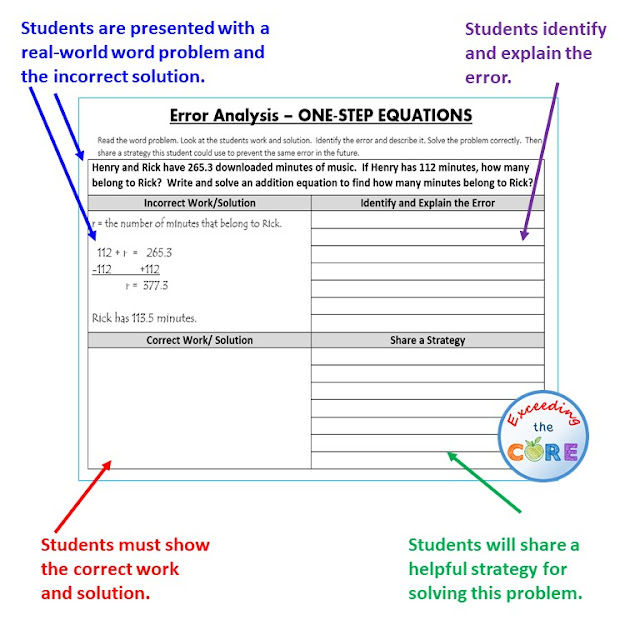One-Step Equations Error Analysis Activity

Each worksheet presents students with a real-word problem. Students must then organize the information using a problem-solving graphic organizer. Students are prompted to identify the important information in the word problem, solve, justify their work and explain their solution.

Each task card has a real-world word problem. Students can work on these cards individually, with partners or in groups.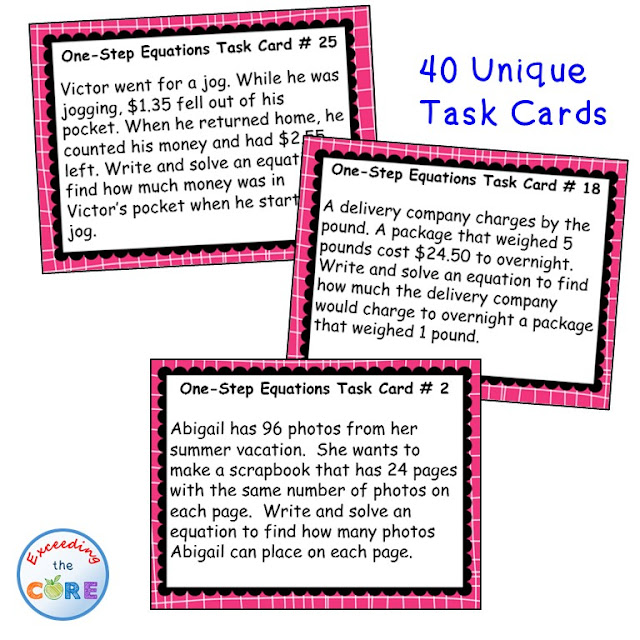One-Step Equations Word Problem Task Cards

Each worksheet includes two sections, Skills Practice and Problems Solving. The SKILLS PRACTICE section of each worksheet includes 6 computational problems to help students sharpen their skills. The WORD PROBLEM section of each worksheet allows students to apply their understanding of the topic to solve 4 real-world problems.One-Step Equations Skills Practice and Problem Solving Activity

A maze, riddle and coloring page is also included. All 3 of these activities allow students to practice this skill while incorporating fun into the classroom! Print or use the digital version of this activity.One-Step Equations Riddle, Maze and Coloring Page

## Teach your students how to add, subtract multiply and divide fractions with these engaging lessons, activities, and assessments.

Explore strategies and resources for the following:

When teaching students operations with fractions it is important to:

✅give your students many opportunities to practice (math stations, homework, classwork, early finisher activities)

✅expose your students to different activities (fun mazes riddles and coloring pages)

✅ have your students apply their understanding by solving real-world word problems (task cards and graphic organizers)

✅ assess your student's understanding (printed or digital assessments)

# Here are a few activities that I include in my Fractions Math Unit.

## Add and Subtract Fractions ActivitiesGraphic Organizers, Error Analysis, Maze, Riddle, Coloring Activity

The Add and Subtract Fractions Unit Resource BUNDLE includes 10 error analysis activities, 10 problem solving graphic organizers, 1 maze, 1 riddle, 1 coloring activity (over 45 skills practice and real-world word problems).

This unit resource bundle includes:

Each page has real-world word problems that is solved incorrectly. Students have to identify the error, provide the correct solution and share a helpful strategy for solving the problem.Add and Subtract Fractions Error Analysis Activity

10 Problem Solving Graphic Organizers - Each worksheet presents students with a real-world problem. Students must then organize the information using a problem-solving graphic organizer. Students are prompted to identify the important information in the word problem, solve, justify their work and explain their solution.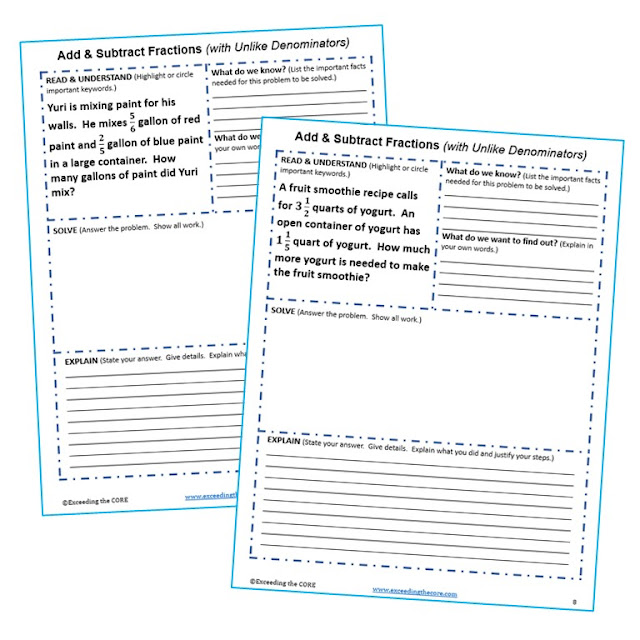Add and Subtract Fractions Problem-Solving Graphic Organizer

A maze, riddle and coloring page is also included. All 3 of these activities allow students to practice this skill while incorporating fun into the classroom!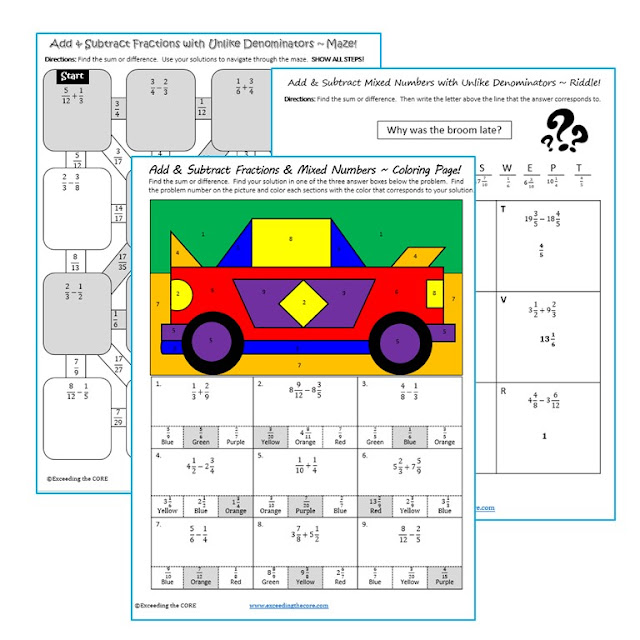Add and Subtract Fractions Maze Riddle and Coloring Page

Topics included:

✔ Add and subtract fractions with unlike denominators.

✔ Add and subtract mixed numbers with unlike denominators.

✔ Rename to find the difference between two mixed numbers.

## MULTIPLY & DIVIDE FRACTIONS BUNDLE

Task Cards, Error Analysis, Word Problems with Graphic Organizers, Maze, Riddle, Coloring Page, Guided Notes, Homework Practice

This Multiply and Divide Fractions UNIT RESOURCE BUNDLE includes 40 task cards, 10 error analysis activities and 10 problem solving graphic organizers, 1 maze, 1 riddle, 1 coloring page activity, 3 guided notes pages, 5 homework practice pages (over 150 skills practice and real-world word problems).

This unit resource bundle includes:

Each page has a real-world word problem that is solved incorrectly. Students have to identify the error, provide the correct solution and share a helpful strategy for solving the problem.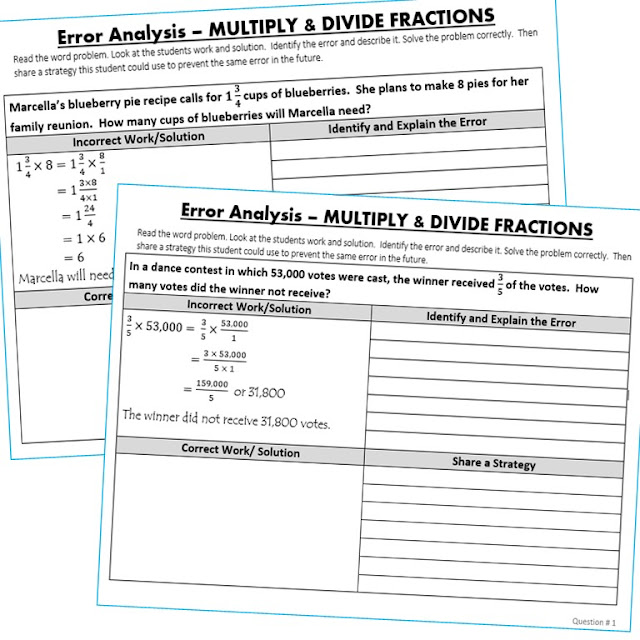Multiply and Divide Fractions Find the Error Activity

Each task card has a real-world word problem. Students can work on these cards individually, with partners or in groups.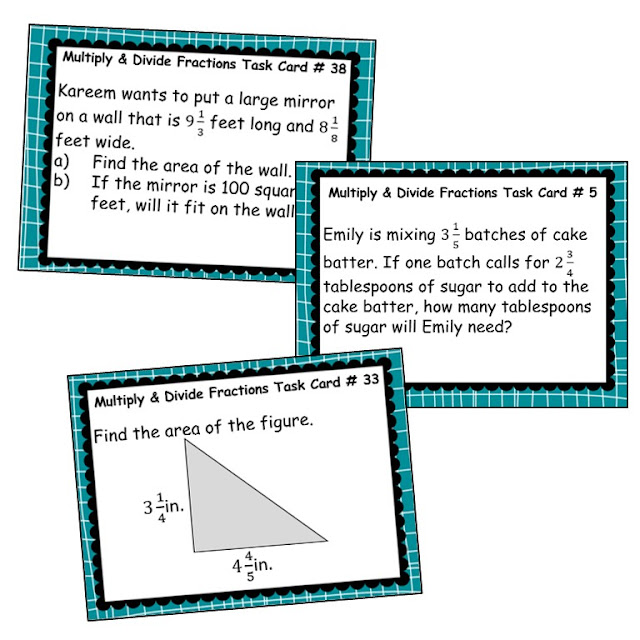Multiply and Divide Fractions Word Problems Task Cards

Each worksheet presents students with a real-world word problem. Students must then organize the information using a problem-solving graphic organizer. Students are prompted to identify the important information in the word problem, solve, justify their work and explain their solution.Multiply and Divide Fractions Word Problem Graphic Organizer

A maze, riddle and coloring page is also included. All 3 of these activities allow students to practice this skill while incorporating fun into the classroom!Multiply and Divide Fractions Maze, Riddle, Coloring Page

These guided notes include essential vocabulary, key concepts and practice questions. Students answer the questions, and then color, doodle or embellish these notes. These guided notes are perfect for interactive notebooks or mini-anchor charts.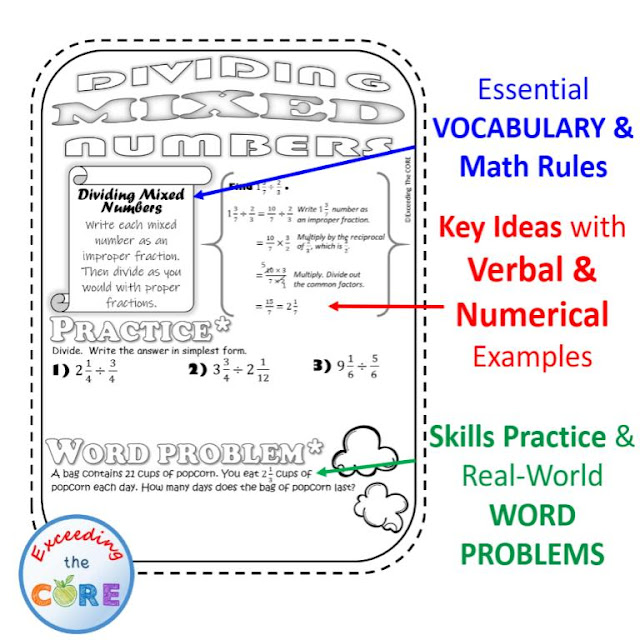Multiply and Divide Fractions Guided Notes

Each worksheet includes two sections, Skills Practice and Problems Solving. The SKILLS PRACTICE section of each worksheet includes 6 computational problems to help students sharpen their skills. The WORD PROBLEM section of each worksheet allows students to apply their understanding of the topic to solve 4 real-world problems.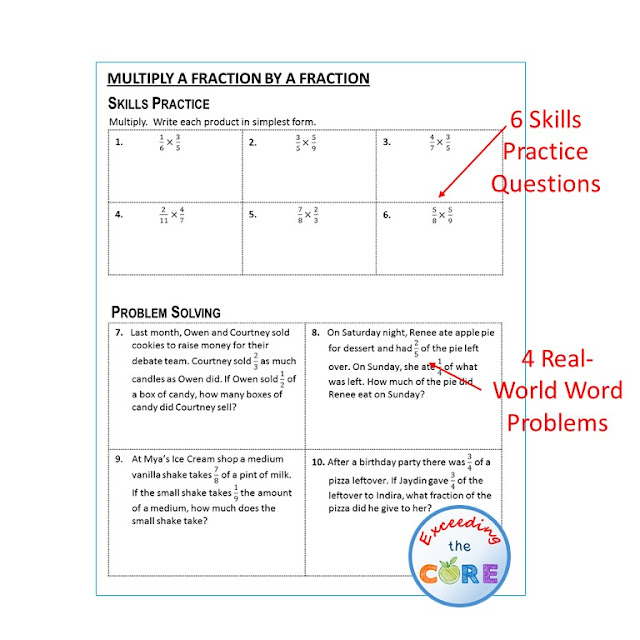Multiply and Divide Fractions Homework Practice Worksheets

## Operations with Fractions Digital Assessments (Google Quizzes)

Use the digital resources in this Operations with Fractions Digital Assessment Bundle to assess your students' understanding of Adding, Subtracting, Multiplying, and Dividing Fractions.

What is Included:

This BUNDLE includes three quizzes. Each quiz includes 10 questions. They are self-grading quizzes and were created with Google Forms. This is perfect for GOOGLE Classroom, remote learning, or distance learning.

Topics Covered:

Benefits of this Resource:

Things to Know:

• This resource was created using Google Forms.
• You and your students must have a free Google account in order to access this activity.
• You can find many more GOOGLE APPS like this to support your remote/online instruction.

Ways to Use this Resource:

• This resource is perfect for homework, computer centers, online testing practice, pre-assessments, post-assessments, RTI (Response to Intervention), and remote / distance learning.

## Benefits of using Google Slides Interactive Activities:

➤ Interactive Activities - Engage students with these DIGITAL and PAPERLESS math activities. Students will use drag and drop, text boxes, and the line tool.

➤ Step-by-Step Instructions- A teacher guide with step-by-step instructions have been included to help you open, share, and use these activities with your students.

.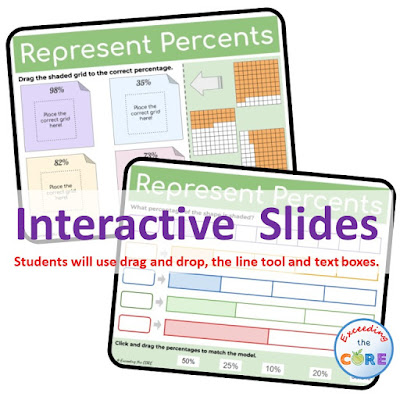Represents Percents Google Slides Activity

Each of the Google Slides bundles shown below can be downloaded from my TpT store.  Once you download the activity, a copy of it will be created in your Google Drive.  Each resource includes the slide activities, answer keys, and teacher directions on how to assign this activity to your students.   Click on the resource to view more details.

## Benefits of using Google Quizzes:

➤Self Grading - After submitting the Google Quiz, the students will receive instant feedback informing them of their scored based on the percent answered correctly and an indication of the problems they got incorrect.

➤Reliable Data - After each assessment is completed, you are able to view responses of individual students as well as frequently missed questions from the entire class.

➤100% Editable - You can add/edit/delete questions, answers, and settings at any time to meet your instructional goals.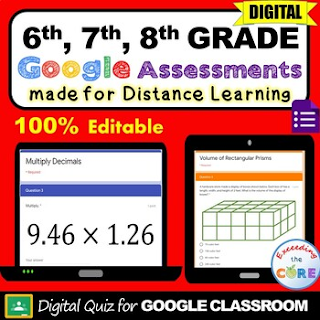This middle school math BUNDLE includes all of my 6th, 7th and 8th Grades Google Quizzes (10 questions per assessment)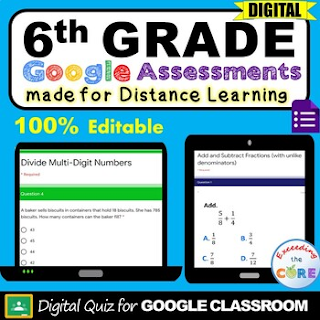Add and Subtract FractionsMultiply FractionsDivide FractionsAdd and Subtract DecimalsMultiply DecimalsDivide DecimalsOperations with DecimalsDivide Multi-Digit NumbersRational NumbersEquivalent ExpressionsOne-Step EquationsSolving Percent ProblemsNets and Surface AreaVolume of Rectangular PrismsClick here to see all of the topics...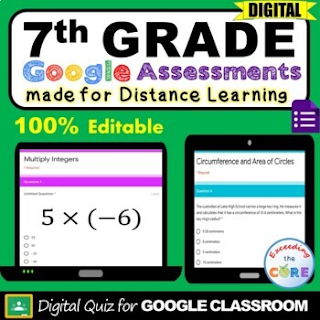Add IntegersSubtract IntegersMultiply IntegersDivide IntegersUnit Rates with FractionsPercent of ChangePercent Problems (Discount, Tax, Mark-up)Simple InterestMultiply using the Distributive PropertyAdd and Subtract Linear ExpressionsFactor Linear ExpressionsTwo-Step EquationsCircumference and Area of CirclesSimple ProbabilityClick here to see all of the topics...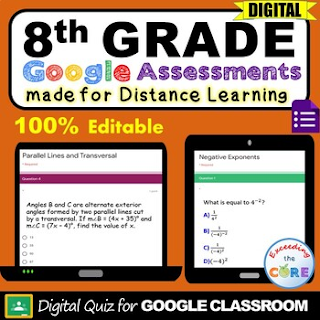Negative ExponentsMultiplication with ExponentsDivision with ExponentsPower RuleScientific NotationMulti-Step EquationsParallel Lines Cut by a TransversalClick here to see all of the topics...